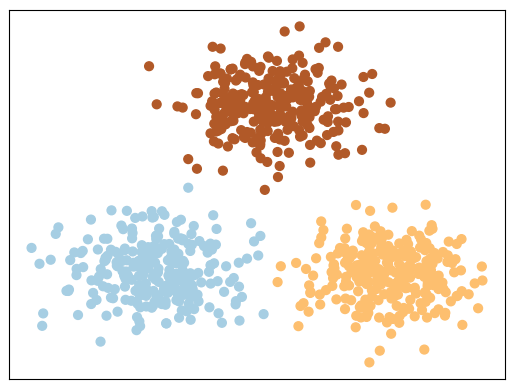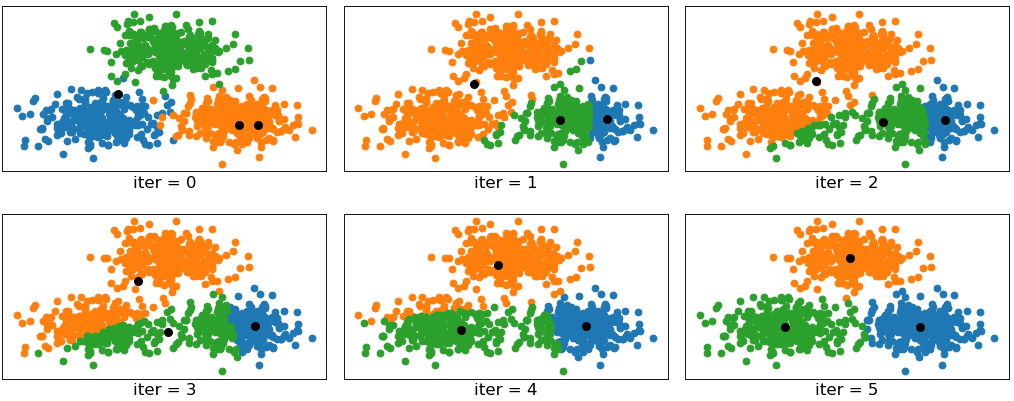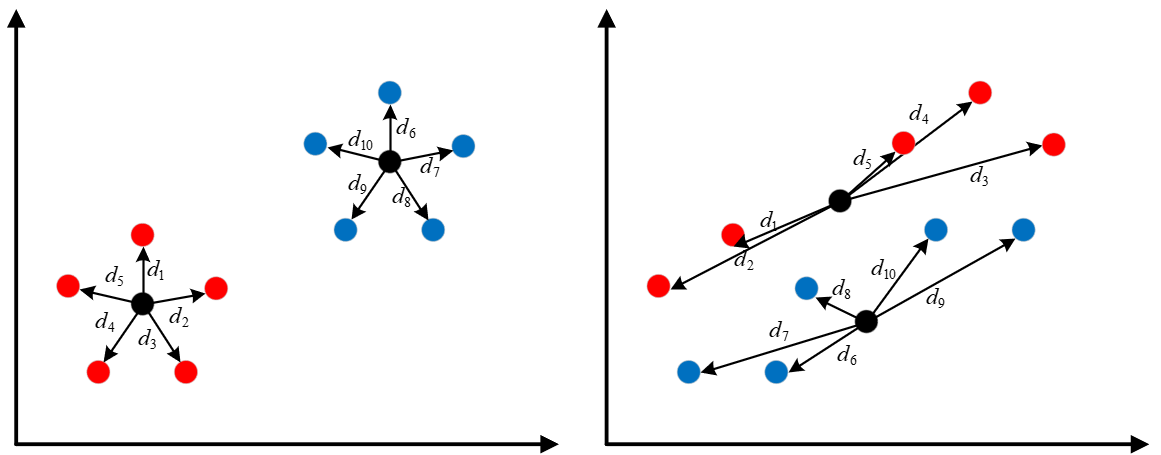# 没有你看不懂的Kmeans聚类算法

## 1 引例## 2 Kmeans聚类

### 2.1 Kmeans算法原理

K m e a n s Kmeans 聚类算法也被称为 K K 均值聚类，其主要原理为：

①首先随机选择 K K 个样本点作为 K K 个簇的初始簇中心；

②然后计算每个样本点与这个 K K 个簇中心的相似度大小，并将该样本点划分到与之相似度最大的簇中心所对应的簇中；

③根据现有的簇中样本，重新计算每个簇的簇中心；

④循环迭代步骤②③，直到目标函数收敛，即簇中心不再发生变化。### 2.3 Sklearn建模

def train(x, y, K):
model = KMeans(n_clusters=K)
model.fit(x)
y_pred = model.predict(x)
nmi = normalized_mutual_info_score(y, y_pred)
print("NMI: ", nmi)

if __name__ == '__main__':
train(x, y, K=3)

# 结果：
NMI:  0.7581756800057784


## 3 Kmeans求解

### 3.1 Kmeans目标函数X = { X 1 , X 2 , . ⋯   , X n } X=\{X_1,X_2,.\cdots,X_n\} 为一个含有 n n 个样本的数据集，其中第 i i 个数据对象表示为 X i = { x i 1 , x i 2 , ⋯   , x i m } X_i=\{x_{i1},x_{i2},\cdots,x_{im}\} m m 为数据对象特征的数目。数据对象分配矩阵 U U 是一个 n × k n\times k 的0-1矩阵（里面只有0和1）, u i p u_{ip} 表示第 i i 个样本被分到第 p p 个簇中。 Z = Z 1 , Z 2 , ⋯   , Z k Z={Z_1,Z_2,\cdots,Z_k} k k 个簇中心向量，其中 Z p = { z p 1 , z p 2 , ⋯   , z p m } Z_p=\{z_{p1},z_{p2},\cdots,z_{pm}\} 为第 p p 个簇中心。则 K m e a n s Kmeans 聚类算法的目标函数可以写为：
P ( U , Z ) = ∑ p = 1 k ∑ i = 1 n u i p ∑ j = 1 m ( x i j − z p j ) 2 (1) P(U,Z)=\sum_{p=1}^k\sum_{i=1}^nu_{ip}\sum_{j=1}^m(x_{ij}-z_{pj})^2\tag{1}

∑ p = 1 k u i p = 1 (2) \sum_{p=1}^ku_{ip}=1\tag{2}

U [ 10 , 2 ] = [ 1 0 1 0 1 0 1 0 1 0 0 1 0 1 0 1 0 1 0 1 ] U_{[10,2]}=\begin{bmatrix} 1 & 0 \\1 & 0 \\1 & 0 \\1 & 0 \\1 & 0 \\0 & 1 \\0 & 1 \\0 & 1 \\0 & 1 \\0 & 1 \\ \end{bmatrix}

## 4 总结

### 引用

 示例代码 ： https://github.com/moon-hotel/MachineLearningWithMe

### 近期文章

原来这就是支持向量机

这就是决策树的思想

朴素贝叶斯算法

K最近邻算法07-022万+
08-15343112-1817万+
05-168万+
07-122万+
06-0191
07-061098
03-113万+
11-121万+
10-2718
02-26615
03-261301
01-153273
03-16144
12-042030
02-15